Скачать презентацию Determine the amount saved if 375 is deposited

6815f4e63f7005e3c45facea76396800.ppt

• Количество слайдов: 8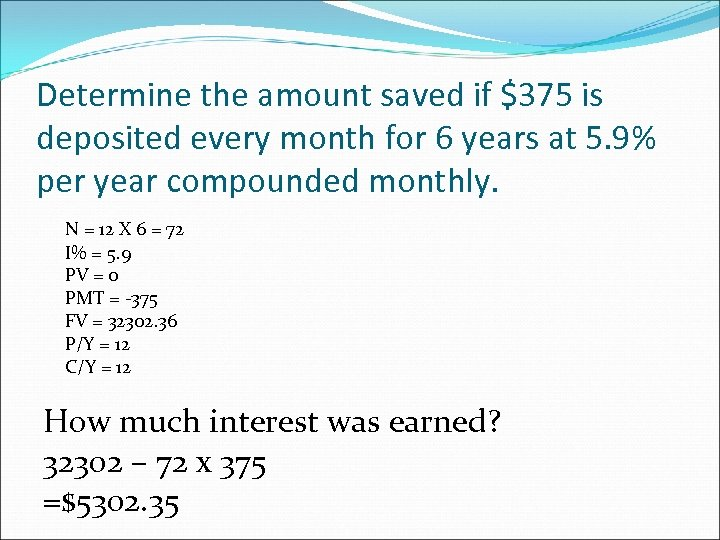Determine the amount saved if \$375 is deposited every month for 6 years at 5. 9% per year compounded monthly. N = 12 X 6 = 72 I% = 5. 9 PV = 0 PMT = -375 FV = 32302. 36 P/Y = 12 C/Y = 12 How much interest was earned? 32302 – 72 x 375 =\$5302. 35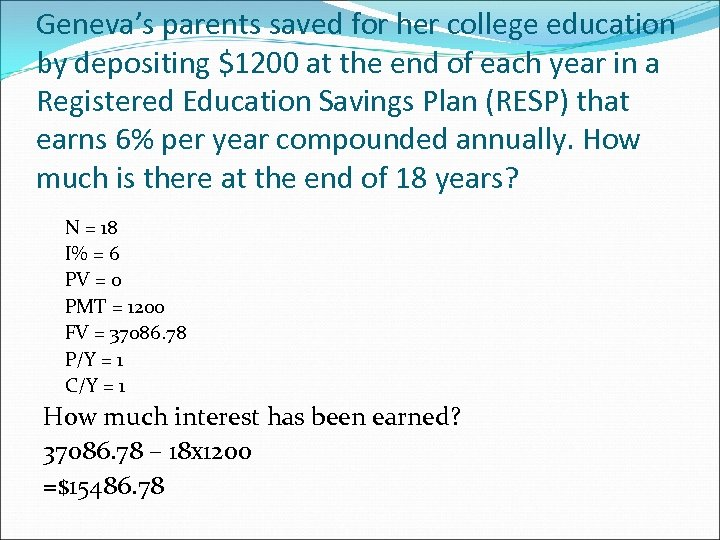Geneva’s parents saved for her college education by depositing \$1200 at the end of each year in a Registered Education Savings Plan (RESP) that earns 6% per year compounded annually. How much is there at the end of 18 years? N = 18 I% = 6 PV = 0 PMT = 1200 FV = 37086. 78 P/Y = 1 C/Y = 1 How much interest has been earned? 37086. 78 – 18 x 1200 =\$15486. 78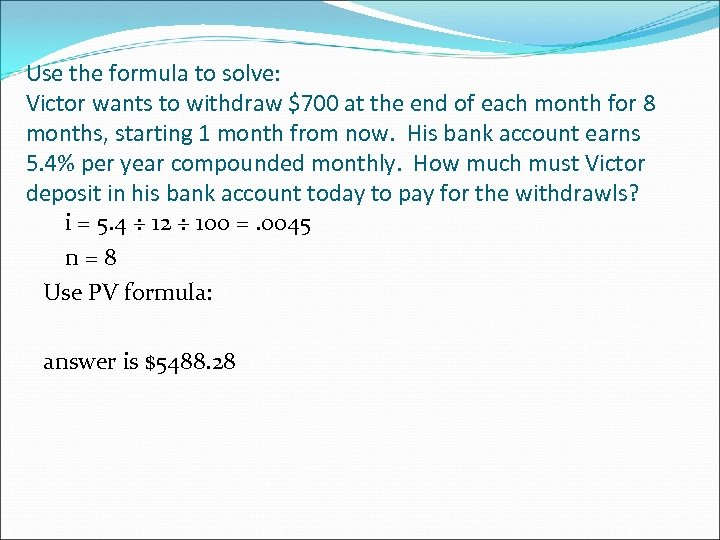Use the formula to solve: Victor wants to withdraw \$700 at the end of each month for 8 months, starting 1 month from now. His bank account earns 5. 4% per year compounded monthly. How much must Victor deposit in his bank account today to pay for the withdrawls? i = 5. 4 ÷ 12 ÷ 100 =. 0045 n=8 Use PV formula: answer is \$5488. 28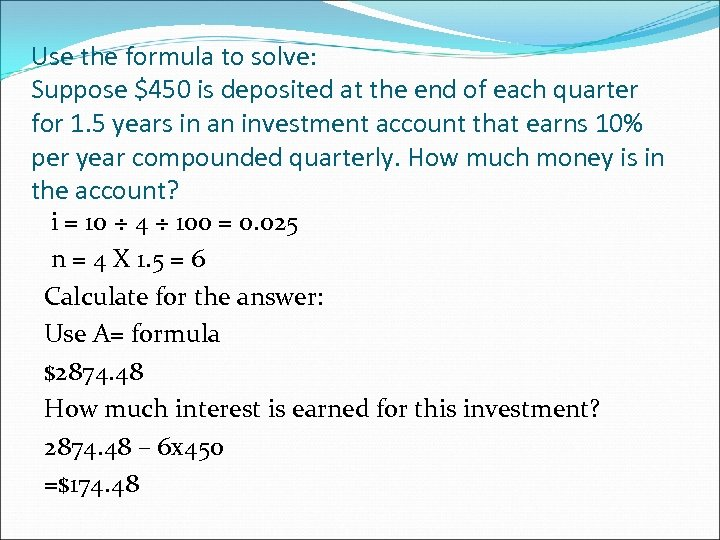Use the formula to solve: Suppose \$450 is deposited at the end of each quarter for 1. 5 years in an investment account that earns 10% per year compounded quarterly. How much money is in the account? i = 10 ÷ 4 ÷ 100 = 0. 025 n = 4 X 1. 5 = 6 Calculate for the answer: Use A= formula \$2874. 48 How much interest is earned for this investment? 2874. 48 – 6 x 450 =\$174. 48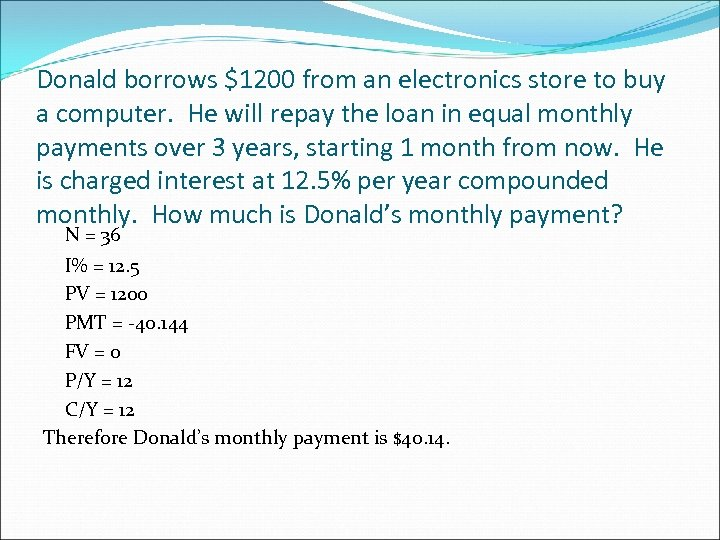Donald borrows \$1200 from an electronics store to buy a computer. He will repay the loan in equal monthly payments over 3 years, starting 1 month from now. He is charged interest at 12. 5% per year compounded monthly. How much is Donald’s monthly payment? N = 36 I% = 12. 5 PV = 1200 PMT = -40. 144 FV = 0 P/Y = 12 C/Y = 12 Therefore Donald’s monthly payment is \$40. 14.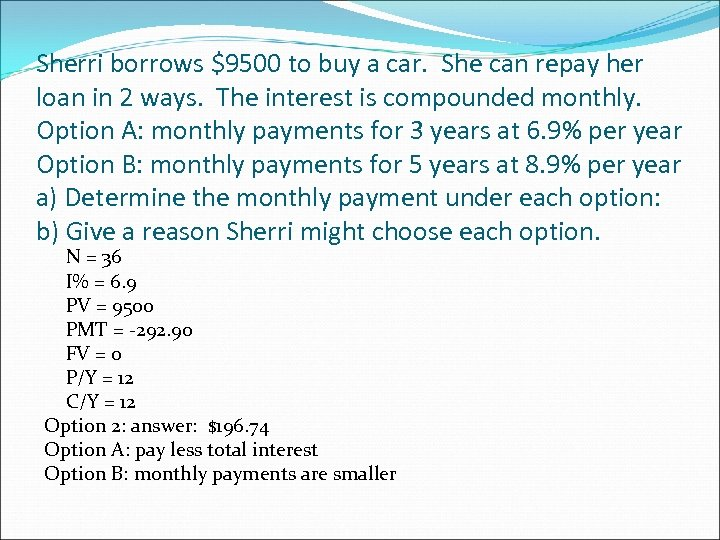Sherri borrows \$9500 to buy a car. She can repay her loan in 2 ways. The interest is compounded monthly. Option A: monthly payments for 3 years at 6. 9% per year Option B: monthly payments for 5 years at 8. 9% per year a) Determine the monthly payment under each option: b) Give a reason Sherri might choose each option. N = 36 I% = 6. 9 PV = 9500 PMT = -292. 90 FV = 0 P/Y = 12 C/Y = 12 Option 2: answer: \$196. 74 Option A: pay less total interest Option B: monthly payments are smaller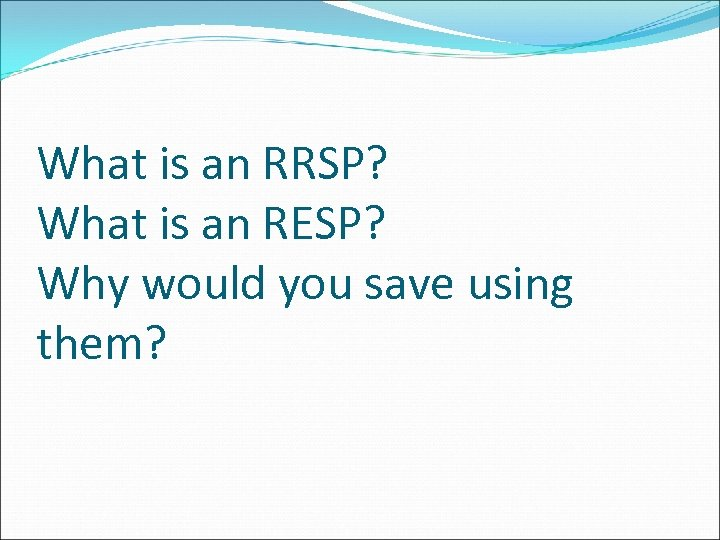What is an RRSP? What is an RESP? Why would you save using them?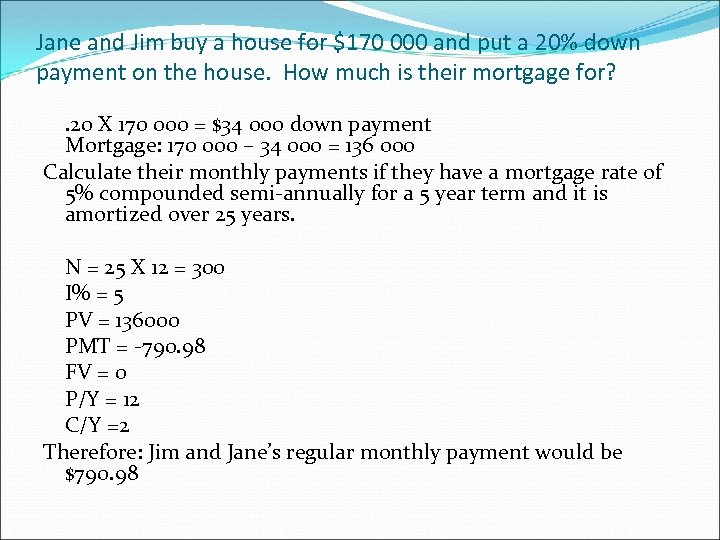Jane and Jim buy a house for \$170 000 and put a 20% down payment on the house. How much is their mortgage for? . 20 X 170 000 = \$34 000 down payment Mortgage: 170 000 – 34 000 = 136 000 Calculate their monthly payments if they have a mortgage rate of 5% compounded semi-annually for a 5 year term and it is amortized over 25 years. N = 25 X 12 = 300 I% = 5 PV = 136000 PMT = -790. 98 FV = 0 P/Y = 12 C/Y =2 Therefore: Jim and Jane’s regular monthly payment would be \$790. 98